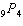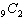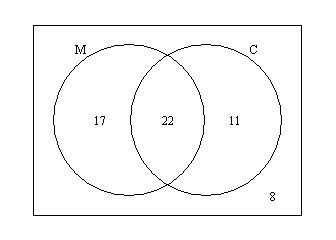Name:    Probability Final Exam Practice Test

Multiple Choice
Identify the choice that best completes the statement or answers the question.

1.

For their uniforms, the Eagles soccer team has a choice of 4 different styles for the shirts, 3 for the shorts and 3 colors of socks. How many different uniforms are possible?
 a. 12 b. 80 c. 40 d. 24 e. 36

2.

How many ways are there to order 3 different items?
 a. 24 b. 6 c. 3 d. 2 e. 5

3.

Evaluatea. 36 b. 15,120 c. 84 d. 126 e. 3,024

4.

Evaluatea. 181,440 b. 9 c. 126 d. 72 e. 36

5.

How many ways are there to choose a president and a vice-president for a school club that consists of 16 members?
 a. 250 b. 232 c. 236 d. 240 e. 238

6.

How many ways are there to choose two representatives for a school club that consists of 13 members to participate in student council meetings?
 a. 74 b. 72 c. 70 d. 78 e. 68

At Paulo’s Pizza, pizzas are available in the following sizes and people buy them with the given probabilities.

 Personal Small Medium Large .08 .21 .32 .39

7.

What is the probability that someone orders a large or a medium pizza?
 a. 0.47 b. 0.6 c. 0.53 d. 0.4 e. 0.71

8.

What is the probability that someone does not order a personal pizza?
 a. 0.79 b. 0.08 c. 0.92 d. 0.61 e. 0.68

9.

Assuming that pizza orders are independent, what is the probability that two consecutive pizza orders are both for a large pizza?
 a. 0.006 b. 0.115 c. 0.152 d. 0.102 e. 0.044

You are going to play 4 games of ping-pong against your best friend. Based on your past experience, you estimate that your probability of winning any single game is .64. It is to be assumed that each game outcome is independent of all other games played.

10.

What is the probability that you win all of the games?
 a. 0.158 b. 0.188 c. 0.107 d. 0.262 e. 0.168

11.

What is the probability that you lose all of the games?
 a. 0.067 b. 0.057 c. 0.047 d. 0.017 e. 0.006

An art class consists of 10th, 11th and 12th grade students. A two-way table, by gender, is given below for the makeup of this class.

12.

If a student from this class is chosen at random, what is the probability that they are in 11th grade?
 a. 0.514 b. 0.486 c. 0.405 d. 0.351 e. 0.243

13.

If a student from this class is chosen at random, what is the probability that they are male?
 a. 0.243 b. 0.405 c. 0.351 d. 0.486 e. 0.514

14.

If a student from this class is chosen at random, what is the probability that they are a female 11th-grader?
 a. 0.189 b. 0.108 c. 0.216 d. 0.162 e. 0.135

15.

If a student from this class is chosen at random, what is the probability that they are a female given that they are 10th-grader?
 a. 0.189 b. 0.135 c. 0.444 d. 0.108 e. 0.162

16.

If a student from this class is chosen at random, what is the probability that they are a 11th-grader given that they are male?
 a. 0.216 b. 0.189 c. 0.162 d. 0.368 e. 0.135

A random sample of 58 adults were surveyed and asked whether they were married and whether they had children. Let M = person is married and C = person has children. The Venn diagram below summarizes their responses.17.

If a person from this survey is chosen at random, what is the probability that they are married?
 a. 0.379 b. 0.293 c. 0.522 d. 0.19 e. 0.672

18.

If a person from this survey is chosen at random, what is the probability that they have children?
 a. 0.379 b. 0.293 c. 0.569 d. 0.419 e. 0.19

19.

If a person from this survey is chosen at random, what is the probability that they have children given that they are married?
 a. 0.379 b. 0.414 c. 0.564 d. 0.293 e. 0.19

20.

If a person from this survey is chosen at random, what is the probability that they are married given that they have children?
 a. 0.293 b. 0.517 c. 0.19 d. 0.379 e. 0.667